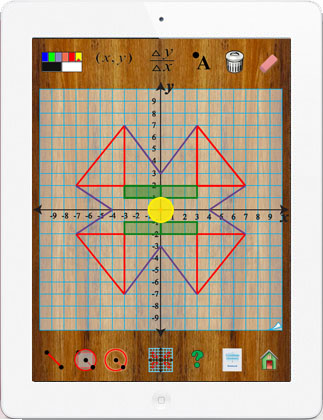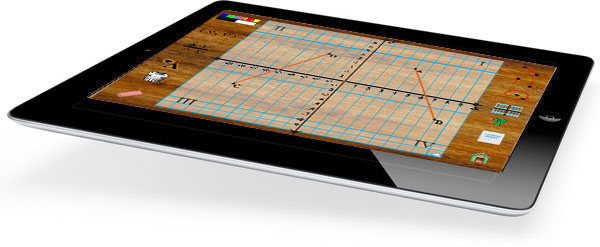Explore Concepts Related to Coordinate Geometry with an Interactive Learning Tool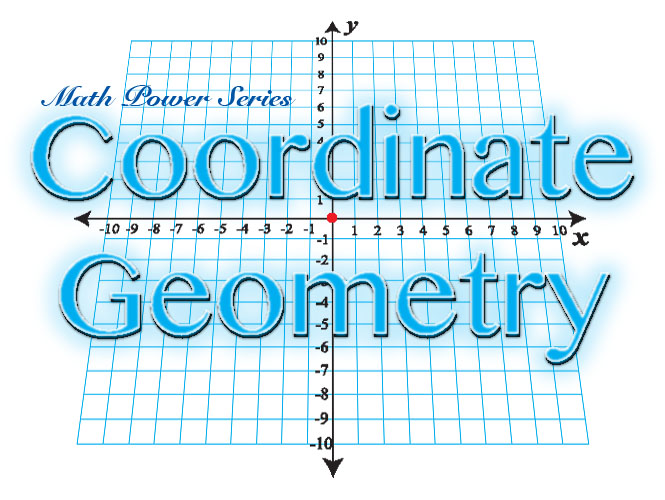The Coordinate Geometry app is a tool designed to help teachers introduce analytic geometry. It provides students with an interactive learning device to help them better understand the system of geometry where the positions of points on a plane are described using ordered pairs. Designed in California by Dr. Fred Ventura, Coordinate Geometry is one of the apps in the Math Power Series. Coordinate Geometry turns your iPad into a mathematical playground for introducing the coordinate plane, ordered pairs, reflection, rotation, translation, slope, y-intercept and other important mathematical concepts.

 Topics Activities Support Materials Coordinate Plane x and y axes Origin (0,0) Coordinate Pairs Quadrants Points, Lines and Planes Slope and y-intercept Dynamically Illustrated Lessons Interactive Inquiry Comprehension Quiz Exploratory Activities iPad App Built-in Instructor's Guide Website

The primary objective for this app is to provide basic information about coordinate geometry through interactive lessons. Tap the notebook icon open illustrated lessons on each of the main topics: Introduction, History of the Coordinate Plane, Slope and y-intercept and Transformations.

Coordinate Geometry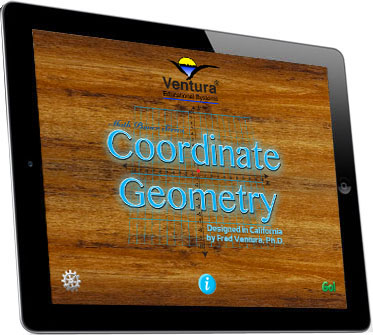The Importance of Coordinate Geometry

The mathematics curriculum traditionally has been designed to provide students with the opportunity to develop skills and acquire knowledge that would be important in adulthood and to prepare sutdents to enter careers that would not require a high level of mathematical ability.

Today the context of mathematics instruction needs to be much broader. The accelerated pace at which modern society produces technological change requires that high school graduates be prepared for careers in qhich mathematics will be very important. In order to be successful in many careers students will need to be confident in their mathematical abilities. They will need to be able to solve problems, to communicate mathematical ideas and to think logically.

Coordinate Geometry turns your iPad into a power tool for teaching about points, lines, planes, transformations, slope and y-intercept. Use Coordinate Geometry to create a presentation while illustrating and discussing the key principles of analytic geometry. Coordinate Geometry is perfect for upper elementary and middle school classrooms. Enrich your mathematics curriculum with the power of Coordinate Geometry!

For many people, coordinate geometry is the familiar in many different ways. For example, in some cities streets are layed out as a coordinate grid... A Street, B Street C Street, intersects with First Avenue, Second Avenue and Third Avenue. The basic concept of coordinate geometry is used in spreadsheets, mapping, and many other systems for locating positions including GPS. In someways it seems that the human brain is intuitively adept at using coordinate systems.

Coordinate Geometry app creates a basic set of learning experiences to help students better understand of the application of coordinate geometry in mathematics. Coordinate geometry bridges algebra and geometry. Equations for example, the standard equation of a line, y = mx + b, defines a line on the Cartesian plane.

Understanding the concepts of slope and intercept are fundamental to success in higher mathematics. Main topics:

• Coordinate Plane
• x and y axes
• Origin (0,0)
• Coordinate Pairs
• Points, Lines and Planes
• Slope and y-intercept

Lessons plans, student activities and online assessments are available as ebooks made for iBooks. Math Fun Coordinate Geometry is an interactive book with lesson strategies, projects for students and assessment tools that are aligned to the Common Core State Standards for Mathematics. Click on the icons below for more information.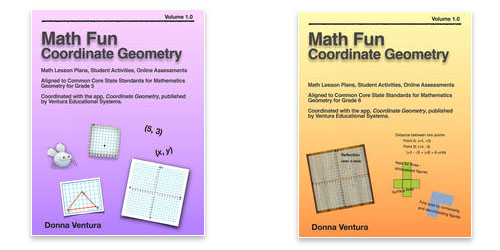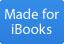Geometry - 5th GradeGeometry - 6th Grade Math Fun Coordinate Geometry Available for iBooks Math Fun Coordinate Geometry Available for iBooks

Coordinate Geometry - A Powerful Teaching Tool

 \$1.99
 Coordinate Geometry Coordinate Geometry is an effective way to introduce points, lines, planes, origin, x-axis, y-axis, slope, intercept, geometric transformation and many other important mathematical concepts. Tap icons and use simple gestures to draw on a coordinate plane. Student interactions with the special tools for exploring coordinate geometry are instantly reflected on the iPad screen. Interactions are described mathematically, for example, tap a point and instantly see and hear the associated coordinate pair. Main Features: Dynamically Illustrated Lessons Interactive Inquiry Comprehension Quiz Exploratory Activities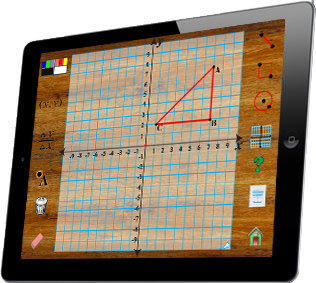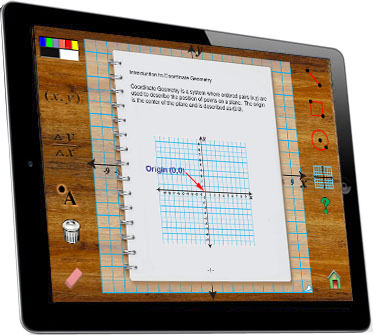Interactive Inquiry Tap areas of the screen to access the special tools for exploring points, lines, planes, origin, x-axis, y-axis, slope, intercept, geometric transformation and many other important mathematical concepts. Learn the terminology that is important to success in geometry by reading illustrated lesons. Coordinate Geometry puts the student in control of the pace and scope of the learning experience. Comprehension Quizzes Review the information presented in the interactive lessons by taking a quiz. The quiz presents randomly generated multiple choice questions for each of the topics. Because the problems in the quiz are randomly generated, each time the quiz is accessed the questions are different.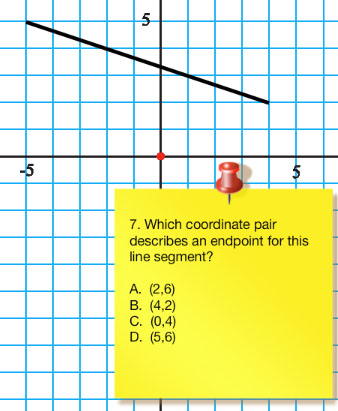Real Learning That Is Fun and Creative In addition to all the math that students can learn using Coordinate Geometry, it is also a tool for just having fun and being creative using mathematics. Let your students develop an intuitive understanding of Coordinate Geometry by letting them play with the app to make designs and patterns. Here’s an idea for a cooperative learning game that you can use if you have two students and two iPads.   Procedure: Two players sit facing each other but can see the other players iPad.   Player 1 draws a figure on his/her iPad.   For example a right triangle at (5,5), (0,0), and (5,0) using black. Player 1 draw the reflection of the figure across the y-axis using red. Player 1 gives verbal directions to player 2 to try to copy the figure and reflection onto his/her iPad. Using math vocabulary player 1 describes the figure and transformation for player 2. When finished the players compare drawings on each iPad.